# What is Analog to Digital Conversion?

Computer NetworkNetworkOperating System

When an analog signal is digitalized, that is known as analog-to-digital conversion.

Consider a human address a voice in the structure of an analog signal. We require to digitalize the analog signal that is smaller inclined to noise. It needed a decrease in the several values in an analog message defined in the digital flow.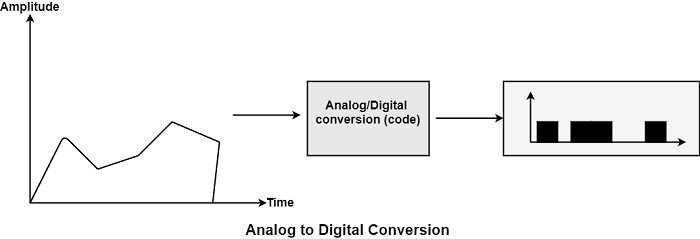In analog-to-digital conversion, the data involved in a constant waveform is modified into digital pulses.

## Methods for Analog-To-Digital Conversion

The various methods for Analog-to-Digital conversion are as follows −

### PAM

The first phase in analog to digital conversion is known as PAM. PAM represents pulse amplitude modulation. This method creates an analog signal, samples it, and creates digital pulses sequences based on sampling. The sampling method used in PAM is more helpful to other manufacturing fields than data communication. PAM is the infrastructure of an essential analog-to-digital conversion method known as pulse code modulation (PCM).

In PAM, the initial signal is sampled at the same intervals, as display in the figure. PAM uses a method known as a sample and hold.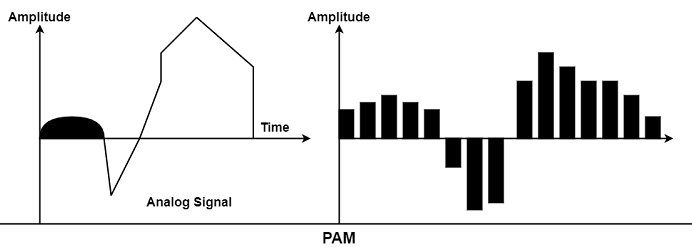### PCM

PCM represents Pulse Code Modulation. PCM method can change the pulses generated by PAM to develop a completely digital signal. To manage this, PCM first measures the PAM pulses. Quantization is a technique of authorizing integral values in a particular area to sampled instances. The outcome of quantization is shown in the figure.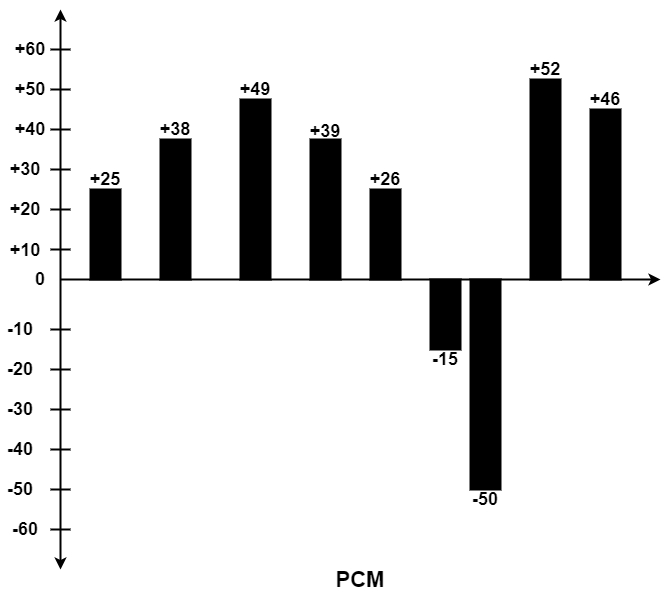The figure displays a simple method of creating sign and magnitude costs to quantized samples. Each cost is interpreted into a six-bit binary proportionate. The seventh bit denoted the sign.

Quantizing using sign and magnitude.

+25 0011001 +39 0100111 -50 1110010
+38 0100110 +26 0011010 +52 0110100
+49 0110001 -15 1001111 +46 0101110

Where sign bit for + is 0 and for – is 1.

### Sampling Rate

As shown in the previous figure, the efficiency of any digital recreation of an analog signal depends on the several samples taken. Using PAM and PCM, we can recreate the waveform precisely by creating infinite samples, or we can make the barest generalization of its direction of change by creating three samples of +24, +48, and +50.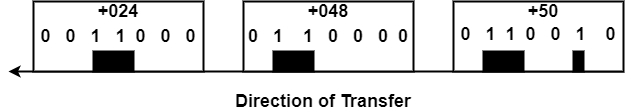From analog signal to PCM digital code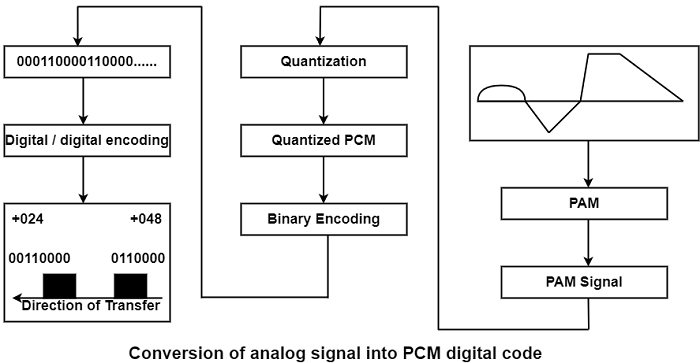Example 1 − What sampling rate is required for a signal with a bandwidth of 9000 Hz (1000 to 10,000 Hz)?

Solution

The sampling rate should be trice the largest frequency in the signal −

Sampling rate = 2 (10, 000) = 20, 000 sample/seconds.

How many Bits per sample?

After discovering the sampling rate, we need to determine the number of bits to be transmitted for each sample. This is based on the method of precision required. The number of a bite are selected, including the original signal can be recreated with the desired accuracy in amplitude.

Bit Rate − After discovering several bits per sample, we can evaluate the bit rate using the following formula −

Bit rate = Sampling Rate x Number of bits per sample

Example 2 − We require to digitize the human voice. What is the bit rate considering eight bits per sample?

Solution

The human voice includes frequencies typically from 0 to 4000 Hz. So the sampling rate is −

Sampling Rate = 4000 x 2 = 8,000 samples/second

The bit rate can be calculated as given below −

Bit rate = Sampling rate x Number of bits per sample

Bit rate = 8000 x 8 = 64,000 bits/s = 64 Kbp